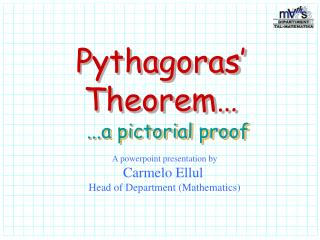# Pythagoras’ Theorem… - PowerPoint PPT PresentationDownload PresentationPythagoras’ Theorem…

Pythagoras’ Theorem…Download Presentation## Pythagoras’ Theorem…

- - - - - - - - - - - - - - - - - - - - - - - - - - - E N D - - - - - - - - - - - - - - - - - - - - - - - - - - -
##### Presentation Transcript

1. Pythagoras’ Theorem… ...a pictorial proof A powerpoint presentation by Carmelo Ellul Head of Department (Mathematics)

2. 2 x 2 4 48 + 48 100 6 6 x 8 8 62 = +36 82 = + 64 100 8 x 6

3. Conclusion… We can therefor say that: In any right angled triangle, the area of the square on the hypotenuse is equal to the sum of the areas of the squares on the other two sides.

4. Conclusion… We can therefor say that: In any right angled triangle, the area of the square on the hypotenuse is equal to the sum of the areas of the squares on the other two sides. RESTART ENDSHOW